## Double Differentiation – Derivation:

Two R-C coupling networks in cascade separated by an ampli­fier A is shown in Fig. 29.36 (a). The amplifier A is to operate as a linear amplifier and the output impedance must be small with respect to the impedance of R2 and C2 so that this combination does not load the amplifier. Let R1 be the parallel combina­tion of R and the input impedance of the am­plifier. The time constants R1C1 and R2C2 must be small in com­parison to the period of the input wave to en­able the circuit to per­form Double Differentiation.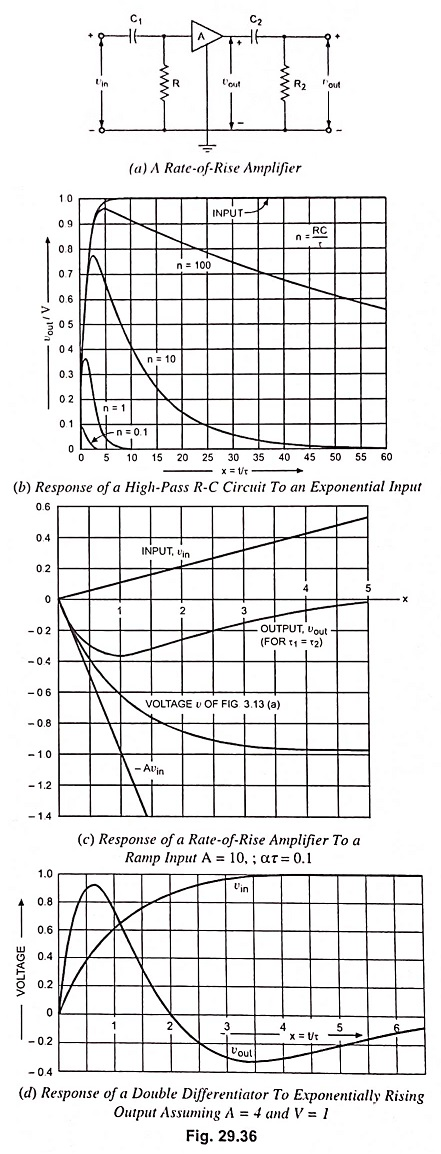When the input is a ramp (vin = αt) of long duration, the output of the inverting amplifier is the negative of the wave­form shown in Fig. 29.10 (b) and is given by equation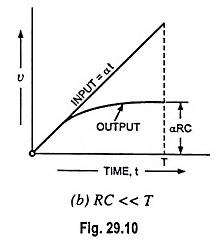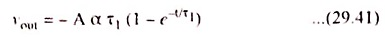where A is the magnitude of the amplifier gain and τ1 is the time constant and is equal to R1C1. This exponential input to the net­work R2C2 in turn gives an output given by Eq. (29.16)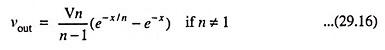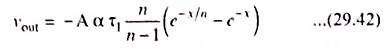If n # 1 where n ≡ τ21, τ2 = R2C2 and x ≡ t/τ1, values of -vout/Aατ1 are plotted in Fig. 29.36 (b) for values of n equal to 0.1, 1.0, 10 and 100. For n = 1, the output is given by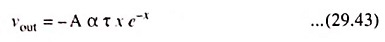This special case is plotted in Fig. 29.36 (c).

The noteworthy point is that a ramp voltage has been con­verted into a pulse. The initial slope of the output wave is the initial slope of the input multiplied by the amplifier gain. This is the reason that the stage in Fig. 29.36 (a) is known as “rate-of­-rise-amplifier”. For a single R-C circuit, the initial rate of change of output is equal to the initial rate of change of input independently of the time constant. Obviously, the same con­clusion can be arrived at for multiple differentiation. A direct check can be made from Eq. (29.42). where it is found that at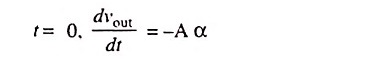As a second illustration of Double Differentiation, we may consider the exponential waveform vin = V(1 – e-t/τ) applied to the circuit shown in Fig. 29.36 (a). For

τ1 = τ2 = τ

or R1C1 = R2C2 = τ, the output is found to be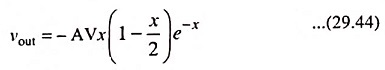where x = t/τ. This result is plotted in Fig. 29.36 (d). The initial slope of output voltage vout is -AV/τ since V/τ is the initial slope of input voltage vin. Also, direct integration reveals that the out­put waveform has as much area above the time axis as below, a fact of importance in some practical problems, such as in pulse spectrometry.

Scroll to Top